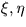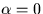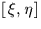Next: SLA_DVDV - Scalar Product
Up: SUBPROGRAM SPECIFICATIONS
Previous: SLA_DTT - TT minus UTC

## SLA_DV2TP - Direction Cosines to Tangent Plane

ACTION:
Given the direction cosines of a star and of the tangent point, determine the star's tangent-plane coordinates (double precision).

CALL:
CALL sla_DV2TP (V, V0, XI, ETA, J)

GIVEN:

 D(3) V direction cosines of star V0 D(3) direction cosines of tangent point

RETURNED:

 D XI,ETA tangent plane coordinates (radians) J I status: 0 = OK, star on tangent plane 1 = error, star too far from axis 2 = error, antistar on tangent plane 3 = error, antistar too far from axis

NOTES:
1.
If vector V0 is not of unit length, or if vector V is of zero length, the results will be wrong.
2.
If V0 points at a pole, the returnedwill be based on the arbitrary assumption thatat the tangent point.
3.
The projection is called the gnomonic projection; the Cartesian coordinatesare called standard coordinates. The latter are in units of the distance from the tangent plane to the projection point, i.e. radians near the origin.
4.
This routine is the Cartesian equivalent of the routine sla_DS2TP.Next: SLA_DVDV - Scalar Product
Up: SUBPROGRAM SPECIFICATIONS
Previous: SLA_DTT - TT minus UTC

SLALIB --- Positional Astronomy Library